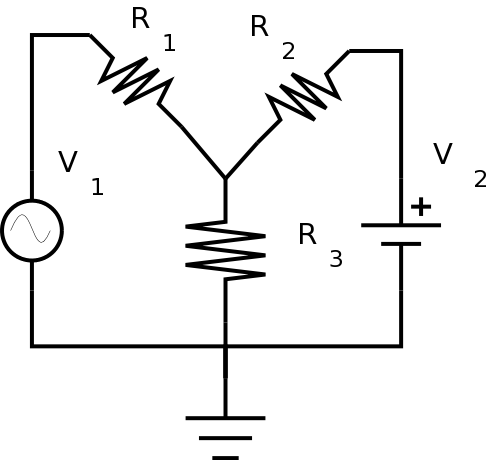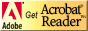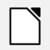# PC221 Analog Electronics I

## Simple AC Circuits

### Objectives

1. To introduce analysis of simple AC circuits.
2. To review the use of the function generator.
3. To introduce measurement of simple AC circuits.
4. To expand the use of SPICE circuit simulation to include transient analysis and the use of cursors.
5. To compare results of analysis, measurement, and simulation.

### Equipment

• digital multimeter, bench power, oscilloscope
• various resistors

### Background

Analyzing circuits with both DC and AC components is slightly more complicated than analyzing DC circuits. To analyze a circuit like this, you need to use the principle of superposition. In other words, you can look at the DC and AC components of the signal in the circuit separately, and then simply add the results.

(Important note: Superposition works for voltage and current but not power, since power is non-linear in either current or voltage.)

#### Determining DC and AC components using Kirchhoff's Laws

Here's how to do it:

1. For the DC analysis,
• replace all AC sources with no DC offset with a wire.
• replace all AC sources with non-zero DC offset with a DC voltage of the offset.
2. For the AC analysis,
• replace all DC sources with a wire.
• replace all AC sources with an AC voltage of the amplitude.

#### Measuring DC and AC components using an oscilloscope

When using the oscilloscope to measure, you can follow a similar process.

1. For the DC analysis:
• Set the coupling for each channel to DC.
• When measuring an AC voltage, only measure its midpoint; not the top or bottom.
2. For the AC analysis:
• With the coupling for each channel to DC, measure the amplitude of any waveform. Ignore its DC offset.
3. Alternatively, for the AC analysis:
• Set the coupling for each channel to AC.
• Measure the amplitude of any waveform.
Recall what the limitations are on measuring voltages using AC coupling. Only use AC coupling if you can't get the AC portion of the signal on the screen at a size that is big enough to measure. Use DC coupling if possible.

#### Measuring DC and AC components using a digital meter

When using the digital meter to measure, you can follow a similar process.

1. For the DC analysis:
• Set the scale to measure DC voltages.
2. For the AC analysis:
• Set the scale to measure AC voltages.
Remember to pay attention to whether the digital meter gives amplitude, peak-to-peak, or RMS values for readings.

#### Determining DC and AC components using SPICE

Doing a SPICE simulation involves two steps as well. Note that you only have to draw the circuit once; you will use different types of analysis to get the DC and AC results. It goes like this:

1. For the DC information:
• Do a DC operating point analysis, as before.
2. For the AC information:
• Do a transient analysis analysis which will show voltages and currents over time, just like the oscilloscope.
##### LTspice Instructions
In LTspice, to see the signals over time use a transient analysis.
##### CircuitLab Instructions
In CircuitLab, to see the signals over time use a time domain analysis.
Hint: To do math with signals, like on a scope, you can use "Add expression" to add, subtract, etc.

### Procedure

#### Setup

1. You'll be modifying the circuit from last week, replacing the DC source for V1 with a 1V 1kHz AC sine wave source with a DC offset of 5V, like this:Leave the other values as before; i.e.
• V2 = 12V
• R1 = 2.7 kΩ
• R2 = 4.3 kΩ
• R3 = 1.2 kΩ

2. Measure the resistor values and the DC supply voltage using the digital multimeter and enter them in the Table 1a:

Quantity Table 1a: Component Values R1 R2 R3 V2

3. Connect the function generator to the oscilloscope. Make sure the scope channel is in DC mode for this. Adjust the wave generator to produce a 1V 1kHz sine wave, with a DC offset of 5V.

4. Connect the circuit as shown. Use the oscilloscope to see that the output of the wave generator does not change significantly when the circuit is connected.

When using a wave generator, always check the output with a scope before connecting it to a circuit. Then when you connect it to the circuit, if it changes drastically, there is probably something wrong so you can disconnect it and examine the circuit.

Put the measured values for V1 using the oscilloscope into Table 1b:

Frequency (from wave generator) Table 1b: Component Value F V1

#### Circuit Measurements: Oscilloscope

1. Measure each of the voltages using the oscilloscope.

(Note: If you're ever adding or subtracting on the oscilloscope, make sure that the coupling and the scale are the same for both channels.)

Sketch the waveform for each of the voltages you measure. Indicate both the amplitude and offset of each voltage in the sketch. Fill in the values in the tables.

Quantity Table 2: DC Values VR1 VR2 VR3 Isupply1 Isupply2 IR1 IR2 IR3

Quantity Table 3: AC Values VR1 VR2 VR3 Isupply1 Isupply2 IR1 IR2 IR3 Frequency

2. Using the measured voltages, calculate each of the currents. Fill in the values in the tables.

3. Measure the period of the waveform, and calculate the frequency. Add it to the table.
Show your results to the lab instructor.

#### Circuit Measurements: Digital Meter

1. Now measure each of the quantities using the digital multimeter.

Note that the multimeter has both AC and DC scales. You'll need to use both scales to measure the voltages to see the AC and DC components of the voltages.

If the digital meter gives RMS measurements, then add a column to the table to show the values converted to amplitude, so they can be compared directly to the other values.(This applies to the current calculations as well.)

2. Using the voltages measured using the digital multimeter, calculate each of the currents.

3. Measure the frequency of the waveform.
Show your results to the lab instructor.

#### Circuit Simulation: Introducing transient analysis

Like last week, you only need to use one of the simulators; either LTspice or Circuitlab, not both.

SPICE can do many different types of circuit analysis.

##### LTspice Instructions
1. Do an operating point analysis, using the measured component values like last week.

Use the information in the tutorial to do a transient analysis. You can also look at pages 8 to 10 of Intro to LTspice . This will show the signals over time, much like what you would see on an oscilloscope.
How to use cursors:
See the information on page 53 of the LTspice manual to learn how to use cursors.

If you use the same scales on your sketch and on your LTspice trace, it will make it much easier to see how they compare.

Show your results to the lab instructor.

2. Save your LTspice files for future use.

##### CircuitLab Instructions
1. Do a DC analysis, using the measured component values like last week.

Do a time domain analysis. This will show the signals over time, much like what you would see on an oscilloscope.

Hint: To do math with signals, like on a scope, you can use "Add expression" to add, subtract, etc.

If you use the same scales on your sketch and on your CircuitLab trace, it will make it much easier to see how they compare.

Show your results to the lab instructor.

2. Save your CircuitLab circuit for future use.

#### Analysis: Kirchhoff's Laws

You can complete the rest of this after you have left the lab. If your results so far are consistent, doing the analysis should be straightforward.
1. Redraw the circuit in both ways (for DC and AC analysis), and analyze them using Kirchhoff's laws, as explained above.

(Use the measured component values like last week.)

(Hint:One of the drawings should look very familiar.)
Remember that for the AC analysis, the resulting voltages and currents will be AC quantities.
Show your two drawings and your results to the lab instructor before continuing.

2. Perform the DC analysis and fill in the appropriate column of Table 2.
Calculate the DC voltage at the top of R3, the DC currents from each supply, and the DC currents through each resistor.

3. Perform the AC analysis and fill in the appropriate column of Table 3.
Calculate the AC voltage at the top of R3, the AC currents from each supply, and the AC currents through each resistor.
Show your results to the lab instructor.

#### Comparing Results

1. Compare the values from the actual circuit to the values from your analysis and measurements above.
The important thing is to rationalize your different results. In other words, if any two quantities differ by more than 20% or so, you should recheck your various values because there is probably a mistake somewhere. For AC values, make sure to state all values using the same terminology; ie. amplitude, peak-to-peak, or RMS. You may have to convert some in this case.
Show your results to the lab instructor.
2. Save your circuit for future use.

Before you leave the lab, have the lab instructor sign your lab notebook immediately after your last entry.

## ResourcesIf you need to update a browser, you might try Firefox which is
• free
• open source
• available for several platformsSince this page uses cascading style sheets for its layout, it will look best with a browser which supports the specifications as fully as possible.

If you are looking for an office package, with a word processor, spreadsheet, etc., you might try LibreOffice which is
• free
• open source
• available for several platformsGo to the main page for the Department of Physics and Computer Science.

Wilfrid Laurier University